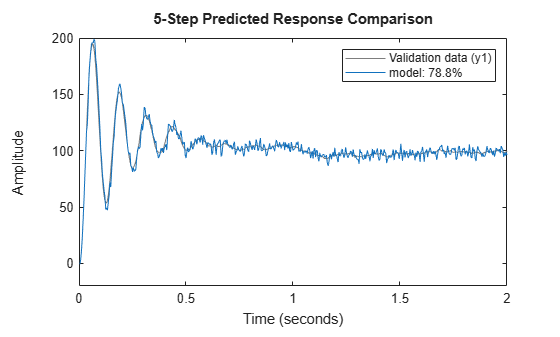# Estimate ARIMA Models

This example shows how to estimate autoregressive integrated moving average (ARIMA) models.

Models of time series containing non-stationary trends (seasonality) are sometimes required. One category of such models are the ARIMA models. These models contain a fixed integrator in the noise source. Thus, if the governing equation of an ARMA model is expressed as A(q)y(t)=Ce(t), where A(q) represents the auto-regressive term and C(q) the moving average term, the corresponding model of an ARIMA model is expressed as

`$A\left(q\right)y\left(t\right)=\frac{C\left(q\right)}{\left(1-{q}^{-1}\right)}e\left(t\right)$`

where the term $\frac{1}{1-{q}^{-1}}$ represents the discrete-time integrator. Similarly, you can formulate the equations for ARI and ARIX models.

Using time-series model estimation commands `ar`, `arx` and `armax` you can introduce integrators into the noise source `e(t)`. You do this by using the `IntegrateNoise` parameter in the estimation command.

The estimation approach does not account any constant offsets in the time-series data. The ability to introduce noise integrator is not limited to time-series data alone. You can do so also for input-output models where the disturbances might be subject to seasonality. One example is the polynomial models of ARIMAX structure:

`$A\left(q\right)y\left(t\right)=B\left(q\right)u\left(t\right)+\frac{C\left(q\right)}{\left(1-{q}^{-1}\right)}e\left(t\right)$`

See the `armax` reference page for examples.

Estimate an ARI model for a scalar time-series with linear trend.

```load iddata9 z9 Ts = z9.Ts; y = cumsum(z9.y); model = ar(y,4,'ls','Ts',Ts,'IntegrateNoise', true); % 5 step ahead prediction compare(y,model,5)```Estimate a multivariate time-series model such that the noise integration is present in only one of the two time series.

```load iddata9 z9 Ts = z9.Ts; y = z9.y; y2 = cumsum(y); % artificially construct a bivariate time series data = iddata([y, y2],[],Ts); na = [4 0; 0 4]; nc = [2;1]; model1 = armax(data, [na nc], 'IntegrateNoise',[false; true]); % Forecast the time series 100 steps into future yf = forecast(model1,data(1:100), 100); plot(data(1:100),yf)```If the outputs were coupled ( `na` was not a diagonal matrix), the situation will be more complex and simply adding an integrator to the second noise channel will not work.